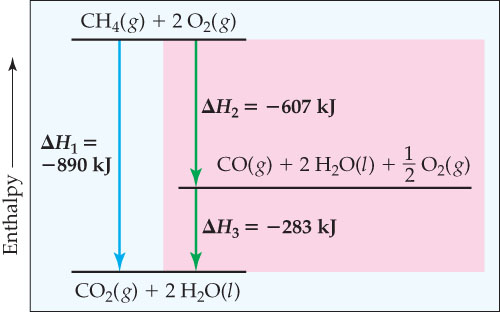# Problem: Enthalpy diagram illustrating Hesss law. The net reaction is the same as in Figure 5.21 in the textbook, but here we imagine different reactions in our two-step version. As long as we can write a series of equations that add up to the equation we need, and as long as we know a value for H for all intermediate reactions, we can calculate the overall H.Suppose the overall reaction were modified to produce 2H2O(g) rather than 2H2O(l). Would any of the values of H in the diagram stay the same?

🤓 Based on our data, we think this question is relevant for Professor Deshmukh's class at UCSD.

###### FREE Expert Solution

We're asked to determine whether any of the values of H in the diagram would stay the same if the overall reaction were modified to produce 2H2O(g) rather than 2H2O(l).

We're given the enthalpy diagram for the overall reaction with the products: CO2 (g) and H2O (l).

Recall that by using Hess's law, we can determine the enthalpy change of an overall reaction by taking the sum of the enthalpies of individual steps.

The individual steps from the enthalpy diagram are as follows: (tip of arrow points to products)

1. CH4 (g) + 2O(g) → CO2 (g) + 2H2O (l)                       ΔH1 = -890 kJ
2. CH4 (g) + 2O2 (g) → CO (g) + 2H2O (l) + ½ O(g)       ΔH2 = -607 kJ
3. CO (g) + 2H2O (l) + ½ O(g) → CO2 (g) + 2H2O (l)     ΔH3 = -283 kJ###### Problem DetailsEnthalpy diagram illustrating Hesss law. The net reaction is the same as in Figure 5.21 in the textbook, but here we imagine different reactions in our two-step version. As long as we can write a series of equations that add up to the equation we need, and as long as we know a value for H for all intermediate reactions, we can calculate the overall H.

Suppose the overall reaction were modified to produce 2H2O(g) rather than 2H2O(l). Would any of the values of H in the diagram stay the same?